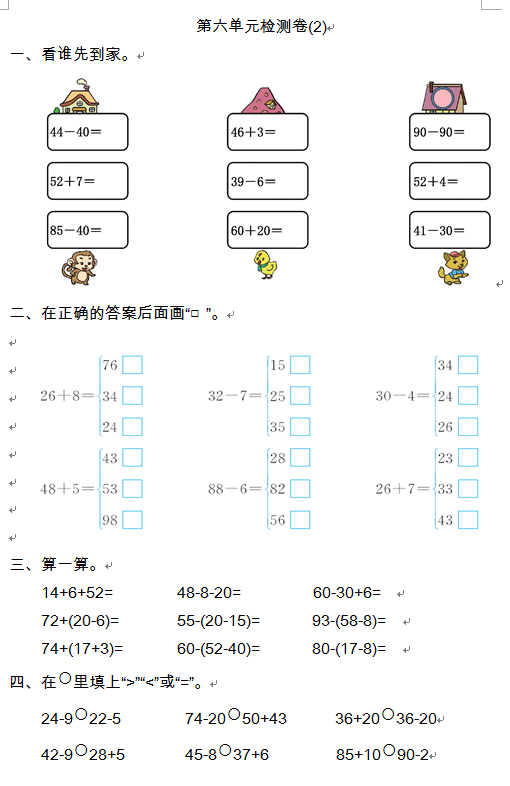14+6+52=　　　　48-8-20=　　　　 60-30+6=

72+(20-6)=        55-(20-15)=        93-(58-8)=

74+(17+3)=       60-(52-40)=        80-(17-8)=

【DOC文档4页】小学人教版一年级数学下册-第六单元检测卷（2）含答案 A4电子版资料_可直接打印_会员免费下载评论信息